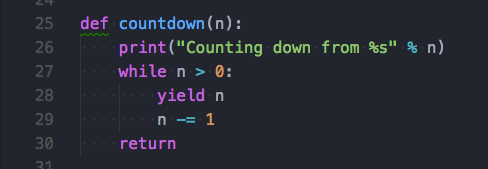# 聊聊Python中晦涩的生成器（yield）与生成器表达式## 什么是生成器？

``````def countdown(n):
print("Counting down from %s" % n)
while n > 0:
yield n
n -= 1
return

c = countdown(10)
print(c.__next__())#python3中的特殊方法，应该避免使用特殊方法
print(next(c))#使用内置函数next()
for i in c :
print(i,end=' ')
``````

``````def countdown1(n):
print("Counting down from %s" % n)
while n > 0:
yield n*n
n -= 1
return

c = countdown1(10)
print(next(c))
print(next(c))
print(next(c))
for i in c :
print(i,end=' ')
``````

## 生成器表达式

``````print('')
print("生成器表达式:")
b = (x*x for x in range(10) if (x % 2 ==0))
print(next(b))
print(next(b))
for i in b :
print(i, end=' ')

``````